MzSpectralFlux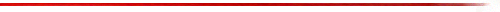### SYNOPSIS

MzSpectralFlux -- Estimates note onsets from changes in spectral magnitudes.
Spectral flux is a measurement of the change in magnitude between frames in a spectrogram. This plugin calculates estimated note onsets from spectral flux and also demonstrates the various steps taken to calculate the spectral flux and derived onset times.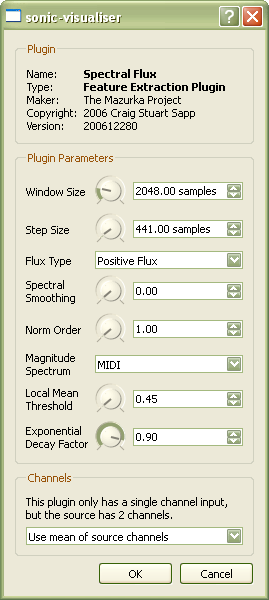### INPUT PARAMETERS

MzSpectralFlux accepts 8 input parameters:

1. Window Size
The size of the audio analysis window in samples for calculating underlying spectra. Window sizes under 1024 samples do not seem to be useful for calculating the spectral flux. Larger window sizes do seem to reduce some noise due to beating of partials in pitched music.
2. Step Size
The number of samples between analysis window starting points. The peak-finding algorithm is currently optimized to a step size of 10 milliseconds which corresponds to 441 samples when the sampling rate is 44100 Hz. Probably not useful to alter this value since some of the peak finding parameters are not yet adjustable by the user.
3. Flux Type
How to process the spectral difference values to generate a flux value:
• "Total Flux" = use all spectral bin slopes.
• "Positive Flux" = set all negative bin slopes to zero.
• "Negative Flux" = set all positive bin slopes to zero.
• "Difference Flux" = non-negative positive minus negative flux values.
• "Composite Flux" = a commixture of the first three flux types: (positive - negative) / (|total - positive|).
4. Spectral Smoothing
The amount of smoothing applied to the spectral frames before the spectral difference is calculated. 0.0 = no smoothing, 1.0 == infinite smoothing. A value of 0.99 usually works very well.
5. Norm Order
The p-value for calculating the norm of the spectral difference vector.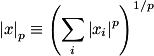Using p=1 generally gives the best results, but you can try various values to see for yourself. Try a p-value of 1.5, for example. On the right is the mathematical definition for the norm which is used in this plugin, where p is this input parameter and xi are the spectral difference values.
6. Magnitude Spectrum
What type of basis spectrum to use for calculating the spectral slope data.
7. Local Mean Threshold
Used for calculating onset times from the scaled spectral flux function. This is the value above the local maximum of the scaled spectral flux function which a value must achieve in order to be considered a peak. If you have too many false positive onsets, increase this value; if you have too many false negatives (missing real onsets), decrease this value.
8. Exponential Decay Factor
Used for calculating onset times from the scaled spectral flux function. This is the feedback gain for an exponentially decaying function based on the scaled spectral flux function. Scaled flux values which are below the exponential decay function are not considered when searching for peaks. If you have too many false positive onsets, increase this value; if you have too many false negatives (missing real onsets), decrease this value.

### OUTPUTS

MzSpectralFlux generates 6 outputs:

1. Underlying Spectrogram
A spectrogram of the underlying spectral data used to calculate the spectral slope and spectral flux values.
2. Spectral Derivative
A spectrogram displaying the differences between successive spectra (output #1). The slope are also processed with according to the "Slope Selectivity" input parameter. Values in the output are normalized for the visual display enhancement (but not normalized when calculating the spectral flux values).
3. Raw Spectral Flux Function
The basic spectral flux calculated from differences between successive spectral frames. This is the starting data for calculating onset times.
4. Scaled Spectral Flux Function
The same thing as output #3, but the mean (average) value of the points in this function is shifted zero, and the values are also scaled so that the standard deviation of the function is 1.0. This makes the function more amenable to comparisons between different function generation methods, and well as for post processing with a peak selection algorithm.
5. Exponential Decay Threshold
Underlying data used in identifying peaks in the scaled spectral flux function. These values are generated by sending the scaled spectral flux function through an exponential smoothing function to suppress noise in the data after in initial onset (noise usually due to beating partials). The input parameter "Exponential Decay Factor" is used to calculate the rate of decay after a note attack.
6. Local Mean Threshold
Also used to calculate peaks in the scaled spectral flux function. These values are generated from averaging in a limited local area on the spectral flux function and then adding an extra offset parameter set by the user as input to the plugin.

## Spectral flux

Spectral flux is a measure of the change in energy between various frequency bands in a sequence of spectra measured from the audio data.

Spectral flux is calculated in three steps:

1. Calculate a sequence of spectra.
2. Measure the difference between successive spectral bins.
3. Collapse the spectral difference of selected bins (from #2) into a single spectral flux value.

The resulting spectral flux function can then be used to identify onset times for notes in the audio data by searching for peaks in the spectral flux function.

Here are some of the example steps in calculating the spectral flux function. The following figure contains the original waveform in orange. Underneath the waveform is the corresponding spectral flux function in green. And underneath the spectral flux function is a display of step #2 in calculating the spectral flux function -- the difference spectrogram.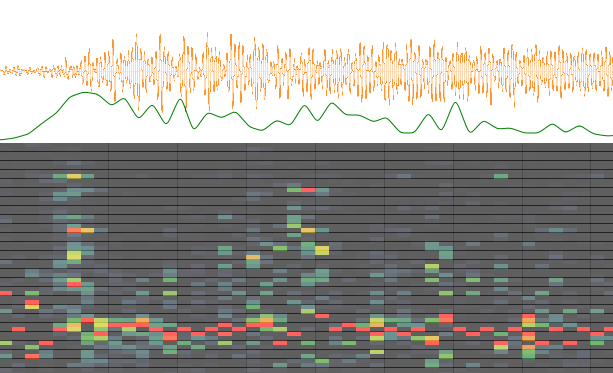## Flux variants

Spectral flux is defined most simply as the Euclidean distance between successive spectral frames: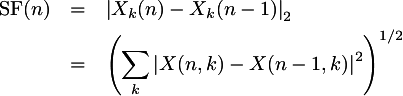This form of spectral flux is a bit noisy due to the equal emphasis on rising and falling spectral energy. If you want to locate note onsets, then you should instead look at only the positive values in the spectral difference: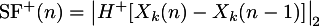Where H+(x) = (x + |x|)/2 is the positive half-wave rectifying function which sets negative values to zero, and leaves positive values unaltered.

There is also negative spectral flux which is usually not interesting by itself: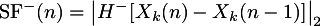where H-(x) = (x - |x|)/2 is the negative half-wave rectifying function which sets all positive values to zero and leaves negative values unaffected.

Note that SF+(n) + SF-(n) = SF(n). This is sometimes interesting to consider, so give it the name difference flux: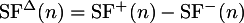Usually it is best if you limit values of SFΔ to non-negative values by setting the negative values to zero: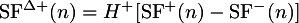And finally, consider the composite flux which is defined as: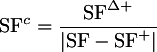This form of spectral flux may be interesting, but is difficult to extract peaks in the same manner as the other types of spectral flux.

Here are visual examples of the first four types of flux. The black curve represents the total flux, the green curve the positive flux, the red curve the negative flux and the blue curve the difference flux.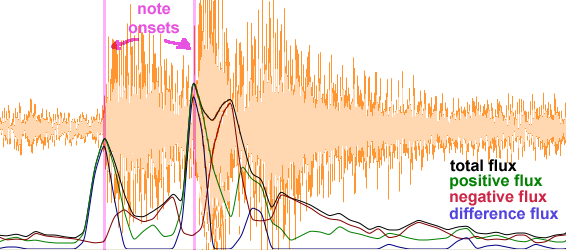### correlation spectral flux

Instead of subtracting adjacent spectral frames to derive a spectral flux value, the change in correlation between three successive spectral frames can be compared: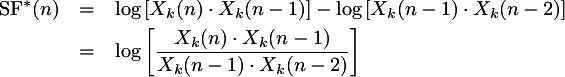Where: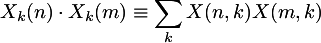is called the dot product, or alternatively unnormalized correlation. Taking the logarithm of the dot product calculations is necessary to have the spectral flux analygous in range to the standard flux definitions which subtract adjacent spectra rather than multiply spectra together.

This method of calculating spectral flux shows potential, but needs some fine-tuning.




### angular spectral flux

The dot product can also be defined as: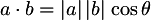It is interesting to look at changes in the angle in isolation rather than changes in the correlation which is a mixture of the angular and magnitude changes.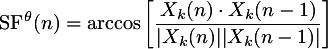Angular spectral flux is also related to subtractive spectral flux as illustrated in the following figure. The current spectral frame can be considered a vector (colored black in the example) as well as the previous spectrum (colored green in the example). The standard definition of spectral flux looks at the changes in the difference between the two spectra which is equivalent to the vector pointing from the previous spectrum to the current spectrum (colored in blue).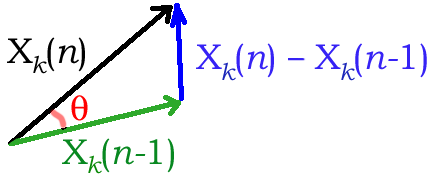Angular flux shows promise, but gives too many false positives in its basic form, and would need to be refined to make it useable for detecting note onsets. Problems to consider: phase wrapping might be causing lots of the noise in this method.

Slightly better peak-to-noise behavior occurs when just using the raw cosine of the angle between the two spectra: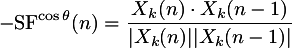(don't know why the negative sign is needed, but it is). Both the angular flux and the cosine flux can generate weird oscillations occasionally during a sustained tone. Fix that problem and they might be useful measures of note onsets...




## L-norm selection

The Euclidean distance used in the previous section definitions of spectral flux is usually not the best method of collapsing the spectral difference values into a single number. In general it is better to just sum the spectral difference values together rather than square each one, then add, then take the square root of the sum: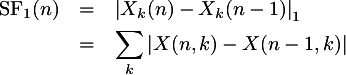In engineering terms, the Euclidean distance is called the L-2 norm, and summation is the L-1 norm, where the norm is defined by the following equation: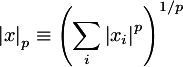where xi is a sequence of numbers to norm, and p is the norm level. For Euclidean distance, or L-2 norm, p=2. For summation, or L-1 norm, p is 1. A generalized equation for the spectral flux using any possible norm would then be: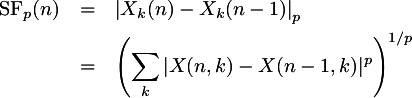In general, the smaller the value of p in the above equation, the better the peak behavior in the spectral flux function. In the following example, the p value is varied to display the differences in the scaled spectral flux function.Notice that lower values of p usually give better resolution of the start of an attack, such as in the second onset identified in the above example.




## Spectral smoothing

Smoothing the spectral frames before calculating the spectral differences can help to remove noise in the spectral flux function. Usually a high amount of smoothing can remove most false-positive detected onsets.

The following figure shows an example of the effect of smoothing on three attacks in a piano recording. Five scaled spectral flux functions are displayed using the following smoothing factors: 0.0 (no smoothing), 0.5, 0.75, 0.9 and 0.99. Notice that the more smoothing that is applied to the spectral frames, the higher the peak at the attack points (purple vertical lines). This allows for a higher local maximum threshold (0.85 in this example) and also a higher exponential decay value (0.95 in this case).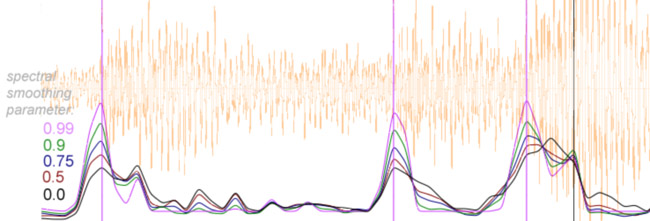Applying spectral smoothing is similar in effect to positive flux calculation. The total flux and positive flux are nearly identical with strong spectral smoothing.




## Peak detection

To estimate note onset times in the spectral flux function, three rules are applied to identify peaks in the spectral flux function which are assumed to correspond to note onsets in the audio:

1. Local maximum: A value in the spectral flux function must be equal to the maximum value in the range of +/- 30 milliseconds (+/- 3 values with a 10 millisecond frame rate).
2. Exponential decay threshold: the test peak must not be less than an exponential decay curve fitted to the spectral flux function. The exponential decay curve is defined as: g[n] = max(f[n], a g[n-1] + (1-a) f[n]), where g[n] is the threshold function, f[n] is the spectral flux function, n is the time index, and a is the exponential decay factor.
3. Local mean threshold: the test peak must be greater than the local mean plus an extra offset factor. The local mean is the average between -90 milliseconds and + 30 milliseconds.

The following example image shows the two threshold functions along with the spectral flux function and the detected onset peaks. The spectral flux function is the thin blue line with the dots at each measured point (10 milliseconds apart). The local mean threshold function is the lower solid curve colored in red. The exponential decay threshold function is the upper solid curve colored in green. Identified onset times are highlighted with purple vertical lines.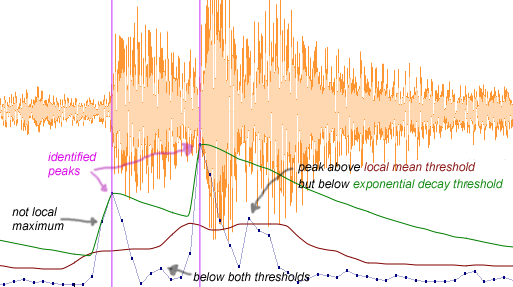Note that there is a fairly large peak after the second onset. While this peak does go above the local mean threshold, it does not get as high as the exponential decay threshold. Therefore it was not identified as an onset peak. Also note the small peak between the first and second detected onsets. While this peak contains a local maximum, it neither rises above the local mean threshold nor meets with the exponential decay threshold, so it is not considered an onset event.

Here is another example of the two threshold functions along with the spectral flux function and detected onsets: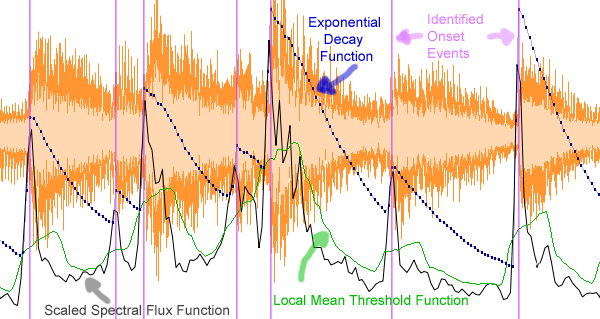### REFERENCES

Dixon, Simon. "Onset detection revisited" in the Proceedings of the 9th International Conference on Digital Audio Effects (DAFx'06). Montreal, Canada; September 18-20, 2006. [Slides]